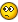## Recommended Posts

Hi All im trying to code an automation script however theres a few variables that require if statements in if statements.. for example current code:

```If \$level = "P" Then
Send(\$type)
sleep(100)
Send(\$city)
Sleep(100)
Send(\$code)
sleep(300)
send("{TAB 3}")
sleep(100)
send(\$level)
sleep(100)
send("{TAB 2}")
sleep(100)
send(\$id)```

now this is where i get stuck because i need another if statement within this if statement to say if \$id = <5 then tab twice else tab once

but i still need the current if because the script requires different actions if \$level is equal to P, S or X

Confused##### Share on other sites

Does this help? Read Language Reference - Conditional Statements in the Help file for a better explanation..

```If something Then
do something
If something else Then
do something else
EndIf
EndIf```
Edited by somdcomputerguy

- Bruce /*somdcomputerguy */  If you change the way you look at things, the things you look at change.

##### Share on other sites

Also need help with a loop within a loop... lol

just saw the reply - okay so does that mean that the

```If something else Then
do something else
EndIf```

does not effect the inital If something then do something, endif? e.g like this.

```1.If something Then
1. do something
within.If something else Then
within.do something else
within.EndIf
can put more stuff here to do with the inital if statement?
EndIf - can change this to:
Elseif \$level = X then
do blahblah```

???

##### Share on other sites

The way I have it written, the second loop will not be processed unless whatever is evaluated in the first loop is true. Maybe you can use a Switch statement on the \$id variable.

- Bruce /*somdcomputerguy */  If you change the way you look at things, the things you look at change.

##### Share on other sites

```If 1+1 = 2 Then

;Do Something

EndIf
;*********************************************************************
;Example 1
If 1+1 = 2 Then

MsgBox(0,"","Expression is equal")

EndIf
;*********************************************************************
;Example 2
If 1+0 = 2 Then

MsgBox(0,"","Expression is equal")

Else

MsgBox(0,"","Expression is not equal")

EndIf
;*********************************************************************
;Example 3
\$BeerInRefrigerator = 0

If \$BeerInRefrigerator = 2 Then

MsgBox(0,"","I have Two beers in refrigerator!")

ElseIf \$BeerInRefrigerator = 0 Then

MsgBox(0,"","I do not have any beer in the refrigerator!")

EndIf
;*********************************************************************
;Example 4

If 1+1 = 2 Then
If 2+2 = 4 Then
If 3+3 = 6 Then
If 4+4 = 8 Then

MsgBox(0,"","All expressions are true")

EndIf
EndIf
EndIf
EndIf```
```\$var = 3

Select

Case \$var = 1
MsgBox(0,"","var = 1")
Case \$var = 2
MsgBox(0,"","var = 2")
Case \$var = 3
MsgBox(0,"","var = 3")
Case \$var = 4
MsgBox(0,"","var = 4")

EndSelect```
```\$CocaColaUnblocksSink = True
\$WaterIsBlue = True

If \$CocaColaUnblocksSink = True Then

MsgBox(0,"1","1",1)
MsgBox(0,"2","2",1)
MsgBox(0,"3","3",1)

If \$WaterIsBlue = True Then

Local \$i = 0

Do
\$i += 1
ConsoleWrite("***=" & "Water is BLUE!" & "=***" & @CRLF)

Until \$i = 5
EndIf

EndIf```

I think is it who you want

Edited by GordonFreeman

##### Share on other sites

got it sorted! thanks guys## Create an account

Register a new account

×

• Wiki

• Back

• #### Beta

• Git
• FAQ
• Our Picks
×
• Create New...